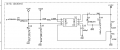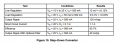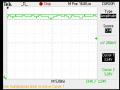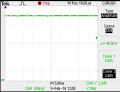# About DC-DC buck converter efficiency calculation

#### Benengineer

Joined Feb 6, 2016
133
Hello, I have DC-DC buck converter in my circuit. I try to test its efficiency and make sure if it meets its datasheet requirement. Here is the circuit.This is converting 24 vols into 3.3 vols DC-DC converter.

Here is the datasheet spec.I measured the output current by measuring voltage of voltage divider. Then I used (3.3-1.24)/8.66k=0.000238mA. My input current is about 32.78mA. So, my input power is about 32.78x24V. My efficiency is Pout/Pin = 0.009%. It totally far away from the datasheet specs.
Thanks

#### ronv

Joined Nov 12, 2008
3,770
Hello, I have DC-DC buck converter in my circuit. I try to test its efficiency and make sure if it meets its datasheet requirement. Here is the circuit.
View attachment 100604
This is converting 24 vols into 3.3 vols DC-DC converter.

Here is the datasheet spec.

View attachment 100605

I measured the output current by measuring voltage of voltage divider. Then I used (3.3-1.24)/8.66k=0.000238mA. My input current is about 32.78mA. So, my input power is about 32.78x24V. My efficiency is Pout/Pin = 0.009%. It totally far away from the datasheet specs.
Thanks
The IC itseld needs some current. That is why they spec it at 500 ma. Add a load to the output and see how it does.
PS what is the IC P/N

•Benengineer

#### SLK001

Joined Nov 29, 2011
1,548
You didn't measure output current at all. You measured the feedback current, which should be very small. Put an 11 ohm and a 22 ohm resistor in series as a load and measure THAT output current. THEN you can calculate the efficiency. At lower currents, efficiency will be lower than at higher currents, so also try putting a 1.1 ohm in series with a 2.2 ohm.

The values that I selected are just for ease of calculations. You can use what you have.

•Benengineer

#### MrAl

Joined Jun 17, 2014
10,075
Hello,

The efficiency calculation you did is obviously wrong or as others have noted something else is wrong like maybe you did not connect a load.

However, once you get it working some care in measuring the input power is needed because it usually is not a smooth waveform but is a pulsing type waveform, or possibly ramping.
In any case it is better to measure the RMS values on the input not the average values. Average values are obtained with most lower cost meters that do not have RMS capability, and even those that do may not be able to respond fast enough for the particular switching frequency.
This means a scope would be better for measring the input power to get a better idea what it really is.

Note the difference between the average power calculated using average values and RMS values for a square wave or other rectangular pulse signal. The average power calculated using the average values of the current and voltage can be way off from the true average power.

#### Lestraveled

Joined May 19, 2014
1,946
OK, look at the data sheet you posted. Look at the output current that the efficiency is stated at, 83.7% at 500 mA. Did you load the output at 500 mA.? No, well load it at 500 mA. and try it again.

Also, @MrAl is right about the current waveform at the input of switching supplies. Very very noisy. While the supply might work just fine with the amount of capacitance you have on your schematic, the lack of capacitance makes measuring the input current problematic. Just to measure the input current, put about 10 times the capacitance just to smooth out the transits into some kind of average reading. It won't be perfect, but it will be better.

•Benengineer

#### Sensacell

Joined Jun 19, 2012
3,180
All switching converters have bias currents that flow regardless of load, this makes them inefficient at low load conditions.
Once you load the output, the bias currents become a small fraction of the total and- the efficiency then shines.

•Benengineer

#### crutschow

Joined Mar 14, 2008
32,043
The 33μF input capacitor should give fairly low ripple voltage at a 500mA output current and the typical switching frequencies of a buck converter so I don't think you need a larger capacitor there.

Actually you want to measure the average value of the input current, since the input power equals the average (not RMS) value of the input current times the input voltage.
This brings up a fallacy in thinking that you always use RMS values where power is concerned.
RMS only applies to the heating power of a current through a resistive load, which is not the case here. The input voltage is DC and the load actually has a negative resistance (i.e. for a given output load, the input current increases with a lower input voltage).

#### MrAl

Joined Jun 17, 2014
10,075
The 33μF input capacitor should give fairly low ripple voltage at a 500mA output current and the typical switching frequencies of a buck converter so I don't think you need a larger capacitor there.

Actually you want to measure the average value of the input current, since the input power equals the average (not RMS) value of the input current times the input voltage.
This brings up a fallacy in thinking that you always use RMS values where power is concerned.
RMS only applies to the heating power of a current through a resistive load, which is not the case here. The input voltage is DC and the load actually has a negative resistance (i.e. for a given output load, the input current increases with a lower input voltage).
Hi,

I can not imagine where you got that idea from, where you can calculate power from average values. Where do you think RMS came from in the first place?

Consider a sine wave with amplitide sqrt(2)::
Vpk=sqrt(2)*sin(2*pi*f*t)

The average voltage of this wave Vavg is 0.9003 volts.
The RMS voltage of this wave Vrms is 1.0000 volts.

Now with a resistance of 1 Ohm...
The average current Iavg is 0.9003 amps.
The RMS current Irms is 1.0000 amps.

The 'power' calculated using average values is Pavg=0.8106 watts.
The power calculated using RMS values is Prms=1 watt.

Now applying 1vdc to the 1 Ohm resistor we get a current of 1 amp and power of 1 watt.

So it becomes clear that we need to use RMS values to get the right power level.

•Benengineer

#### crutschow

Joined Mar 14, 2008
32,043
I can not imagine where you got that idea from, where you can calculate power from average values. Where do you think RMS came from in the first place?

Consider a sine wave with amplitide sqrt(2)::
Vpk=sqrt(2)*sin(2*pi*f*t)

The average voltage of this wave Vavg is 0.9003 volts.
The RMS voltage of this wave Vrms is 1.0000 volts.

Now with a resistance of 1 Ohm...
The average current Iavg is 0.9003 amps.
The RMS current Irms is 1.0000 amps.

The 'power' calculated using average values is Pavg=0.8106 watts.
The power calculated using RMS values is Prms=1 watt.

Now applying 1vdc to the 1 Ohm resistor we get a current of 1 amp and power of 1 watt.

So it becomes clear that we need to use RMS values to get the right power level.
I know where RMS came from. Do you?
RMS cannot be blindly used in all situations.
RMS is the value of voltage that gives the same heating power as a DC voltage into a resistive load. The calculation is derived from the fact that the power in a resistor is proportional to the square of the voltage. It does not necessarily apply to other types of loads.
But now do the calculation for a constant current load (or a negative impedance current load such as buck converter) and tell me what you get.Last edited:

#### MrAl

Joined Jun 17, 2014
10,075
Hello again,

I know where RMS came from. Do you?
RMS cannot be blindly used in all situations.
RMS is the value of voltage that gives the same heating power as a DC voltage into a resistive load. The calculation is derived from the fact that the power in a resistor is proportional to the square of the voltage. It does not necessarily apply to other types of loads.
But now do the calculation for a constant current load (or a negative impedance current load such as buck converter) and tell me what you get.Hello again,

Well why dont YOU do it and then explain what it is you are getting at? Perhaps i dont understand your intention here or something. Constant voltage or constant current may be simpler i agree, but we can not just assume we have either of these.

The calculation for average power is:
Pavg=(1/T)*Integral(i(t)*v(t)) dt

Do you agree with that or no?

Also, if we see a phase angle difference we have to apply that information as well, such as in the power factor calculation.

Last edited:

#### crutschow

Joined Mar 14, 2008
32,043
As I stated, the input to a switching regulator looks like a constant current load with a negative impedance.
I agree with you calculation. If you do the integral for a constant I, the power into the load is Vave*I.
Since I is constant,the power is proportional to the average value of V not the RMS value.

•Benengineer

#### Benengineer

Joined Feb 6, 2016
133
The 33μF input capacitor should give fairly low ripple voltage at a 500mA output current and the typical switching frequencies of a buck converter so I don't think you need a larger capacitor there.

Actually you want to measure the average value of the input current, since the input power equals the average (not RMS) value of the input current times the input voltage.
This brings up a fallacy in thinking that you always use RMS values where power is concerned.
RMS only applies to the heating power of a current through a resistive load, which is not the case here. The input voltage is DC and the load actually has a negative resistance (i.e. for a given output load, the input current increases with a lower input voltage).
Hi Crutschow, thank you for your help. I still don't understand why 33uF input capacitor should give fairly low ripple voltage at a 500mA output current and the typical switching frequencies of a buck converter. If you think this input capacitor value is so big, Is there any formula to get right capacitor value here?

Ben

#### Benengineer

Joined Feb 6, 2016
133
In fact, the swtiching frequencies I measured is about 135.36KHz for the buck.

#### crutschow

Joined Mar 14, 2008
32,043
In fact, the swtiching frequencies I measured is about 135.36KHz for the buck.
The input current for a 24V input, a 3.3V output and 80% efficiency is .5 * 3.3 /24 /.8 = 86mA
A rough calculation of the ripple voltage is Vrip = I / f*C = 86m /(135k*33u) = 19.3mV, a very low input ripple voltage.

•Benengineer

#### Benengineer

Joined Feb 6, 2016
133
The input current for a 24V input, a 3.3V output and 80% efficiency is .5 * 3.3 /24 /.8 = 86mA
A rough calculation of the ripple voltage is Vrip = I / f*C = 86m /(135k*33u) = 19.3mV, a very low input ripple voltage.
Thank you for your help, Crutschow. You are the man. Here is the DC-DC converter output test graph before removed the Zener diode and after removed the Zener diode. Please have a look at it.The DC-DC converter before removed the Zener diodeThe DC-DC output graph after removed the Zener diode
As mentionded the beginning, there is noise sound and you can see the noise above the graph and after removed the Zener diode. Why does the first graph output form that kind of output?
Thanks.

#### Benengineer

Joined Feb 6, 2016
133
Crutschow, can I change 33uF inot 22uF? In this way, we can save more power dissipation than in 33uF. Please let me know.
Thank you.

#### MrAl

Joined Jun 17, 2014
10,075
Hello again,

The best way to calculate the power is with:
Pavg=(1/T)*Integral(i(t)*v(t)) dt

or all bets are off. That is the definition of average power, which we usually just call "power".

The average value of a wave F(t) is:
Vavg=(1/T)*integral(F(t))dt integrated over the period.

Now let's try to calculate the power into a 1 ohm resistor using the average values and using the actual definition of average power for the wave with unspecified frequency f:
F(t)=sqrt(2)*sin(2*pi*f*t)

First using the average values for the voltage:
Vavg=(1/T)*integral(F(t))dt=0.9

and the average values for the current:
Iavg=(1/T)*integral(F(t))dt=0.9

now the power:
P=Vavg*Iavg=0.9*0.9=0.81 watts

Now using the definition of power:
Pavg=(1/T)*integral(F(t)*F(t))dt=1 watt

So the using the definition of power the it came out to 1 watt, and using the average values it came out to only 0.81 watts, so using the average values did not work.

When the voltage or current is constant we have a different situation, but one thing we know for sure is that the defining equation for power Pavg=(1/T)*integral(i(t)*v(t))dt ALWAYS works.

In fact, the only time we can use average value is if one of the waves is constant because then that constant comes out of the integration:
(1/T)*integral(K*F(t))dt=K*(1/T)*integral(F(t))dt=K*Vavg or K*Iavg.

#### crutschow

Joined Mar 14, 2008
32,043
Crutschow, can I change 33uF inot 22uF? In this way, we can save more power dissipation than in 33uF. Please let me know.
Thank you.
The capacitor value has little to do with power dissipation.

#### crutschow

Joined Mar 14, 2008
32,043
Hello again,

The best way to calculate the power is with:
Pavg=(1/T)*Integral(i(t)*v(t)) dt

or all bets are off. That is the definition of average power, which we usually just call "power".

The average value of a wave F(t) is:
Vavg=(1/T)*integral(F(t))dt integrated over the period.

Now let's try to calculate the power into a 1 ohm resistor using the average values and using the actual definition of average power for the wave with unspecified frequency f:
F(t)=sqrt(2)*sin(2*pi*f*t)

First using the average values for the voltage:
Vavg=(1/T)*integral(F(t))dt=0.9

and the average values for the current:
Iavg=(1/T)*integral(F(t))dt=0.9

now the power:
P=Vavg*Iavg=0.9*0.9=0.81 watts

Now using the definition of power:
Pavg=(1/T)*integral(F(t)*F(t))dt=1 watt

So the using the definition of power the it came out to 1 watt, and using the average values it came out to only 0.81 watts, so using the average values did not work.

When the voltage or current is constant we have a different situation, but one thing we know for sure is that the defining equation for power Pavg=(1/T)*integral(i(t)*v(t))dt ALWAYS works.

In fact, the only time we can use average value is if one of the waves is constant because then that constant comes out of the integration:
(1/T)*integral(K*F(t))dt=K*(1/T)*integral(F(t))dt=K*Vavg or K*Iavg.
I don't disagree with anything here.
My point was that you can't use the usual value for RMS AC voltage into a constant-current load and get the correct value of power.
You need to use the average value of the voltage.